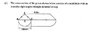# Ext Surface of Prism

risphysics
I'm a new student to complex maths & I'm after the formula to calculate the exterior surface area of the prism - see attached diagram.
I know formula for a surface area of triangular prism = ab + ph and cylinder = 2pir^2 + 2 pi rh. Don't know where/how to apply formula to half of these.

#### Attachments

•figure.jpg
10.2 KB · Views: 278

maze
There are a couple ways to do this. One is to break the surface into a bunch of pieces, like in this image:
http://img371.imageshack.us/img371/3480/areapeicesij5.png [Broken]

and then find the surface area of each of the peices individually and add them up (Have you learned how to do this yet?)

Another way to find the outer surface (excluding the top and bottom peices) is to multiply the perimeter of the base by the height.

Both ways will give the same result.

Last edited by a moderator:
risphysics
No I haven't learned the method of breaking it up into pieces. Could you elaborate further pls.
I'm studying Residential Drafting via correspondece, so unfortunately I do not have a teacher to refer to. Thanks again.

Homework Helper
"Breaking it into pieces" is really just looking at it.

I see the surface as having two rectangular pieces, one long cylindrical piece, and, on the ends, two right triangles and two semi-circles. Find the areas of each of those and add. You are given the length of the long part and the diameter (so twice the radius) of the circular pieces. To find the width of the rectangular pieces, use the Pythagorean theorem on the right triangles.

The area of the surface of a semicircular cylinder is the circumference of the semicircle multiplied by the length of the cylinder. Surely you know how to find the area of a semi-circle, rectangle, and triangle.

maze
Can you please tell me which of the things in this list you know how to do, and which you don't, so I can make a better explanation.
1) Find the area of a rectangle.
2) Find the area of a right triangle (triangle where one of the angles is 90 degrees).
3) Find the length of one side of a right triangle if you know the other 2 sides.
4) Find the area of a circle.
5) Find the perimeter of a circle.

Thanks.

risphysics
Can you please tell me which of the things in this list you know how to do, and which you don't, so I can make a better explanation.
1) Find the area of a rectangle.
2) Find the area of a right triangle (triangle where one of the angles is 90 degrees).
3) Find the length of one side of a right triangle if you know the other 2 sides.
4) Find the area of a circle.
5) Find the perimeter of a circle.

Thanks.
(1) a = bxh
(2) a = 1/2 bxh
(3) AB^2 = AB^2 + (AB)^2
(4) = nr^2 (circ / diam)
(5) Not 100% sure on finding perimter of cirlce

Thanks for taking the time Maze.

maze
If you look at all the surfaces on there from the picture in my previous post, parts 1,2,4,5,6, and 7 are triangles, rectangles, and half-circles, which you know how to find the area of, so I'm not going to talk about those.

That leaves only a single piece left, piece 3, the curving one. Now, this brings up the question: what does it mean to find the area of a curving sheet? It means, if you were going to create the curving part by bending a piece of paper, how much paper would you need?

You can try this with a real piece of paper, or imagine it in your mind, but if you un-bend piece 3 and make it flat, it will be a rectangle. It's height is already given, and the width is one half of the perimeter of the circle.

(By the way, perhaps the word perimeter is confusing you. When I say perimeter, I just mean the circumference of the circle)

The perimeter of the whole circle is 2*pi*r, so the perimeter of one half the circle is pi*r.

risphysics
If you look at all the surfaces on there from the picture in my previous post, parts 1,2,4,5,6, and 7 are triangles, rectangles, and half-circles, which you know how to find the area of, so I'm not going to talk about those.

That leaves only a single piece left, piece 3, the curving one. Now, this brings up the question: what does it mean to find the area of a curving sheet? It means, if you were going to create the curving part by bending a piece of paper, how much paper would you need?

You can try this with a real piece of paper, or imagine it in your mind, but if you un-bend piece 3 and make it flat, it will be a rectangle. It's height is already given, and the width is one half of the perimeter of the circle.

(By the way, perhaps the word perimeter is confusing you. When I say perimeter, I just mean the circumference of the circle)

The perimeter of the whole circle is 2*pi*r, so the perimeter of one half the circle is pi*r.
Thanks so much ... you have simplified it for me to understand now.